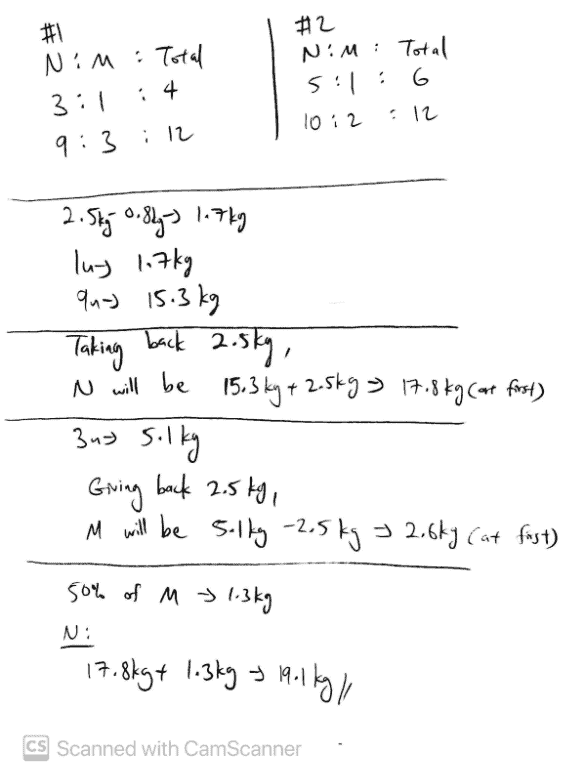# QuestionHi AnikaP

Let me try to help you with this question. First of all, this question makes use of the concept known as Constant Total. This is a situation that happens when 2 or more parties give and take from one another. Their total remains the same among themselves. Hence the name Constant Total.

With that understanding, take a look at my working and their ratios. There are 2 scenarios here and let’s call them #1 and #2.

Notice that in #1, the total units is 4. While in #2, the total units is 6. Since this is a Constant Total question, we must ‘make the total same’ so that we can link the 2 ratios in #1 and #2 together. Hence, the complex form is manipulated to such. Now both scenarios have 12 units in total.

Let us compare #1 and #2. We see that N gives 2.5 kg to M in #1. And in #2, N gives 0.8 kg to M. This leaves 1.7 kg more for N in #2 than in #1. And we can see that the for the end results, in #1, N has 9 units and in #2, N has 10 units. This shows that the 1 unit more that comes from the 10 units compared to the 9 units represents our 1.7 kg.

So therefore we find out that 9 units that represent N in #1 will be 15.3 kg.

So we find out that N has 15.3 kg after after giving 2.5 kg to M. Which means, originally, N has 17.8 kg.

Taking a step back, we can see that in #1, M has 3 units, this represents 5.1 kg. It means that M has 5.1 kg after receiving 2.5 kg from N. So this means that originally, M has 2.6 kg.

To solve the question, 50% of M transferred to N, will be taking 1.3 kg from M, and adding to the 17.8 kg in N.

So, our answer will be 19.1 kg.

Hope this helps! Feel free to PM or text me (:

http://www.futureachievers.com.sg

9114 3397

0 Replies 1 Like ✔Accepted Answer